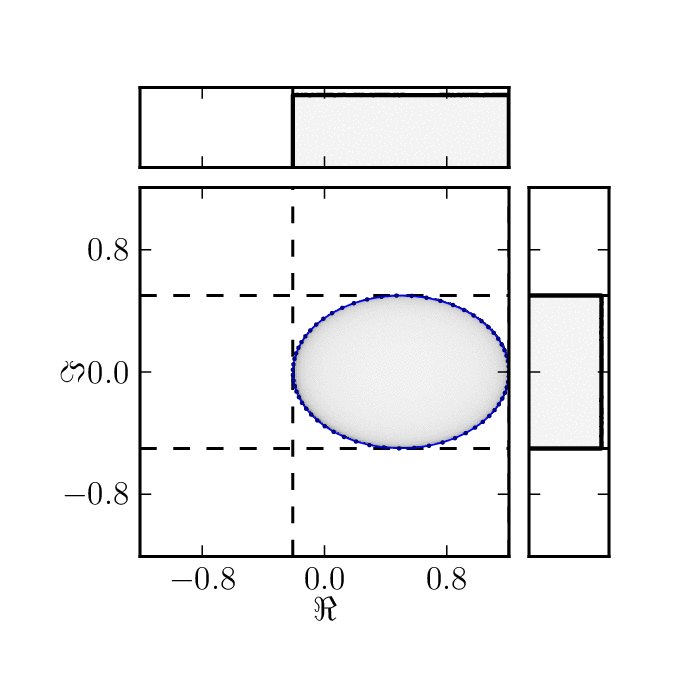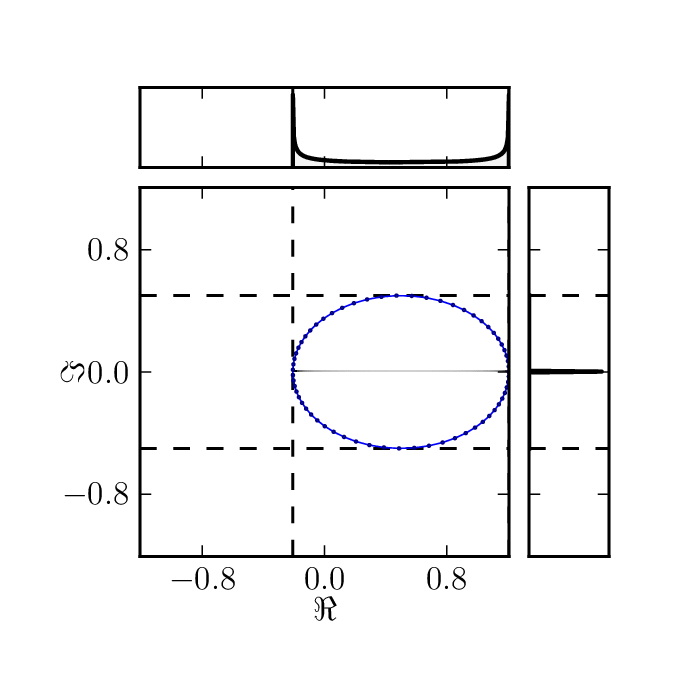Shadows of family of matrices $$M_2(\phi)=e^{i\phi} \begin{pmatrix} 0&1\\ 0&1 \end{pmatrix},$$

with $\phi\in [0, 2\pi ]$. Upper and right plots present marginal distributions of real and imaginary part corresponding to shadows of hermitian and anti-hermitian parts of $M_2(\phi)$.

Standard numerical shadow with respect to complex states.Standard numerical shadow with respect to real states.